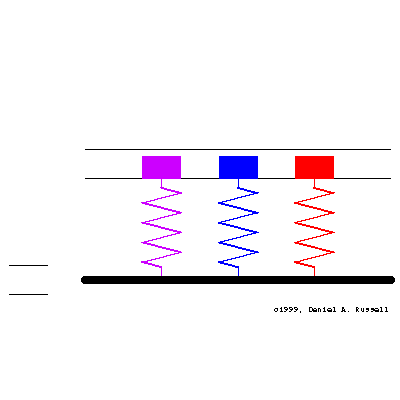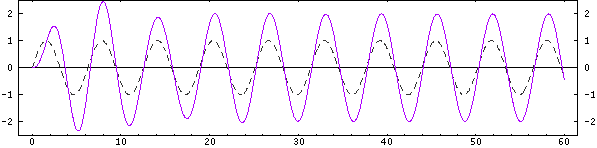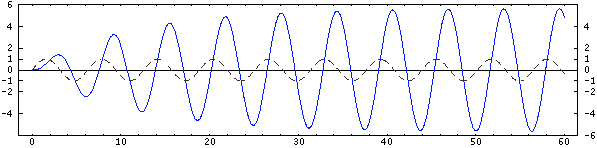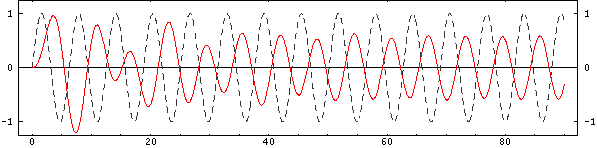Acoustics and Vibration Animations Daniel A. Russell, Ph.D. Graduate Program in Acoustics The Pennsylvania State University All text and images on this page are ©2004-2011 by Daniel A. Russell and may not used in other web pages or reports without permission.# Response of a 1-DOF oscillator to a displacement of its baseThree simple 1-DOF mass-spring oscillators have natural frequencies (from left to right) of f1=1.6, f2=1.0, f3=0.63. At time t=0 the base starts moving with sinusoidal displacement s(t) = S0sin(t) where the driving frequency is f=1.001. The damping rate for all three oscillators is 0.1 so that the initial transient motion decays and a steady-state is obtained. The animation at left shows the motion of the base and the resulting motion of all three oscillators together. The masses are color coded to match the frequencies above and the plots below. The horizontal lines indicate the maximum displacement of the base.

### Plots showing transition from transient to steady state motion

In all three plots below, the dashed gray curve represents the displacement of the base, while the colored curves represent the displacement of the masses.

Mass 1: Below Resonance - positive added mass
The first oscillator is being driven below its natural frequency. Its motion is in-phase with that of the base and its displacement is slightly larger than the base displacement. In terms of the input mechanical impedance as seen by the base, this oscillator provides an apparent positive added mass to the base.Mass 2: apparent damping
The second oscillator is being driven very near resonance. Its displacement lags that of the base by 90° and it grows until steady state is reached. In terms of the input mechanical impedance as seen by the base, this oscillator provides an apparent damping, removing vibrational energy from the base.Mass 3: Above Resonance - negative added mass
The third oscillator is being driven at twice its natural frequency. The transient behavior takes longer to decay. Once steady state has been achieved, the displacement is less than that of the base and it almost 180° out of phase with the base. In terms of the input mechanical impedance as seen by the base, this oscillator provides an apparent negative added mass to the base.Back to the Vibration and Waves Demos Page